• 【R描述统计分析】描述统计量描述统计量中位数百分位数方差标准差变异系数样本校正平方和样本未校正平方和样本极差偏度系数、峰度系数练习 描述统计量 例： #输入体重 X1<-c(35, 40, 40, 42, 37, 45, 43, 37, 44,...
【R描述统计分析】描述统计量描述统计量中位数百分位数方差标准差变异系数样本校正平方和样本未校正平方和样本极差偏度系数、峰度系数练习
描述统计量
例：
#输入体重
X1<-c(35, 40, 40, 42, 37, 45, 43, 37, 44, 42, 41, 39)

#计算体重的均值和标准差
mu1<-mean(X1); sigma1<-sd(X1)

#输入胸围
X2<-c(60, 74, 64, 71, 72, 68, 78, 66, 70, 65, 73, 75)

#计算胸围的均值和标准差
mu2<-mean(X2); sigma2<-sd(X2);

中位数
median(x,na.rm=FALSE)

当 na.rm=TRUE时，可以处理缺失数据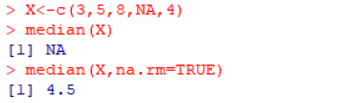百分位数
quantile(x) 提供五个值
quantile(x,probs=seq(0,1,0.25)), na.rm=FALSE,names=TRUE)

产生0%，20%，40%，60%，80%，100%的分位数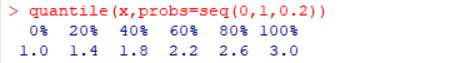方差
var(x)：注意分母为n-1
标准差
sd(x)
变异系数
CV=sd(x)/mean(x)
样本校正平方和
css=sum((x-mean(x))^2)
样本未校正平方和
uss<-sum(x^2)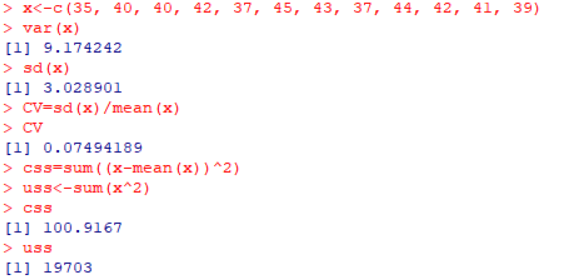样本极差
R=max(x)-min(x)
偏度系数、峰度系数
R的扩展统计程序包fBasics提供：
函数 skewness( ) 用来求样本的偏度
函数 kurtosis( ) 用来求样本的峰度
练习
对于一组数据，编写一组程序，输出这组数据的基本统计量（如：均值、方差、标准差、偏度、斜度、极差、极值等等），并能给出其直方图，密度函数曲线，QQ图。
data_outline <- function(x){
n <- length(x)                                #数列长度
m <- mean(x)                                  #均值
v <- var(x)                                   #方差
s <- sd(x)                                    #标准差
me <- median(x)                               #中位数
cv <- 100*s/m                                 #样本变异系数
css <- sum((x-m)^2)                           #样本校正平方和
uss <- sum(x^2)                               #样本未校正平方和
R <- max(x)-min(x)                            #极差
R1 <- quantile(x,3/4)-quantile(x,1/4)         #上下四分位数之差，又称样本半极差
sm <- s/sqrt(n)                               #样本标准误
g1 <- n/((n-1)*(n-2))*sum((x-m)^3)/s^3        #偏度系数
g2 <- ((n*(n+1))/((n-1)*(n-2)*(n-3))*sum((x-m)^4)/s^4- (3*(n-1)^2)/((n-2)*(n-3)))  #峰度系数
data.frame(N=n, Mean=m, Var=v, std_dev=s, Median=me, std_mean=sm, CV=cv, CSS=css, USS=uss, R=R, R1=R1, Skewness=g1, Kurtosis=g2, row.names=1)
}

在程序编辑完成后，可以输入想要描述的数组，编写程序如：
w <- c(75.0, 64.0, 47.4, 66.9, 62.2, 62.2, 58.7, 63.5, 66.6, 64.0, 57.0, 69.0, 56.9, 50.0, 72.0)
data_outline(w)

得到的结果为：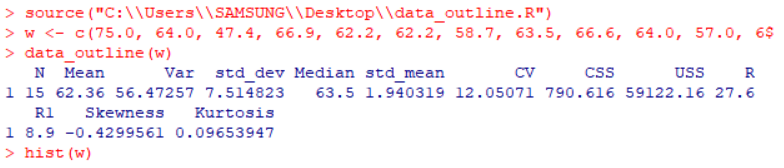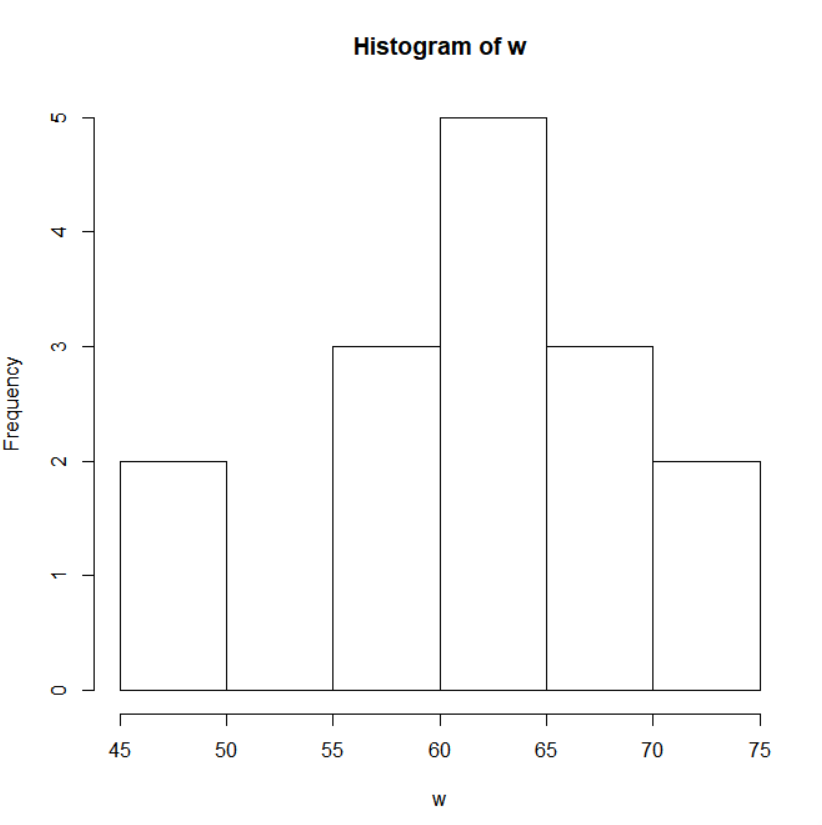展开全文r语言 统计学
• 【R描述统计分析】多元数据
【R描述统计分析】多元数据的数据特征与相关分析二元数据的数据特征及相关系数二元数据的相关性检验Ruben置信区间的近似逼近公式Pearson相关性检验cor.test()使用方法多元数据的数字特征及相关矩阵多元数据的图表示方法轮廓图星图调和曲线图
在R中，计算多元数据的均值与方差采用数据框的结构输入数据，计算比较方便。
二元数据的数据特征及相关系数
例如对矿石中的两种成分进行统计分析：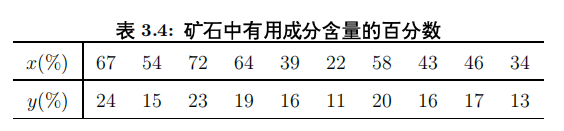ore<-data.frame(
x=c(67, 54, 72, 64, 39, 22, 58, 43, 46, 34),
y=c(24, 15, 23, 19, 16, 11, 20, 16.1, 17, 13)
)
ore.m<-mean(ore); ore.m
ore.s<-cov(ore); ore.s
ore.r<-cor(ore); ore.r

attach(ore)
cor.test(x,y)

cor.test(x,y, method="spearman")

cor.test(x,y, method="kendall")

mean( ) 函数 计算均值
cov( ) 函数 计算协方差
cor( ) 函数 计算相关矩阵（相关系数）
cov.wt 计算加权协方差
cor.test 计算相关性检验
cov(ore) = var(ore)
二元数据的相关性检验
对于二元数据：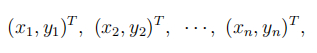可以计算出样本的相关系数r
且总体的相关系数为：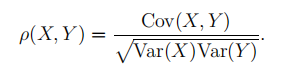当样本的个数n充分大时，样本的相关系数r可以作为总体相关系数的估计，即样本个数较大时，样本相关，总体也相关。
问题是：当样本个数n取到多少时，样本相关才能得到总体相关？
Ruben置信区间的近似逼近公式
Ruben（鲁宾）给出了总体相关系数的区间估计的近似逼近公式
设n是样本个数，r是样本相关系数，u是标准正态分布的上α/2分位点，则计算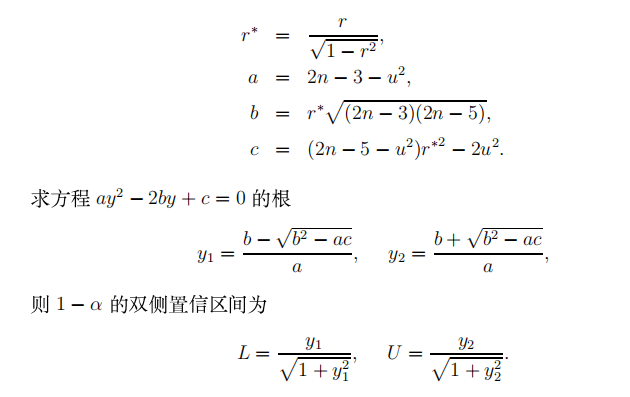按照上述计算公式，编写R程序：
ruben.test<-function(n, r, alpha=0.05){
u<-qnorm(1-alpha/2)
r_star<-r/sqrt(1-r^2)
a<-2*n-3-u^2; b<-r_star*sqrt((2*n-3)*(2*n-5))
c<-(2*n-5-u^2)*r_star^2-2*u^2
y1<-(b-sqrt(b^2-a*c))/a
y2<-(b+sqrt(b^2-a*c))/a
data.frame(n=n, r=r, conf=1-alpha,
L=y1/sqrt(1+y1^2), U=y2/sqrt(1+y2^2))
}

将n,r调入已编好的ruben.test() 函数中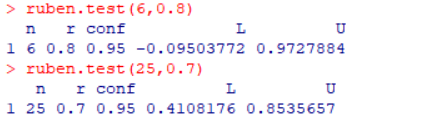①n=6，r=0.8
置信区间为（﹣0.095，0.97），其置信下界是负数，即使r=0.8，也不能说明总体是相关的
②n=25，r=0.7
置信区间为（0.41,0.85），此时基本能说总体是相关的
关于置信区间的近似逼近方法还有David提出的图表方法，Kendall和Stuart提出的Fisher逼近方法等。
Pearson相关性检验
确认总体是否相关最有效的方法是作总体（X,Y）^T 的相关性检验，可以证明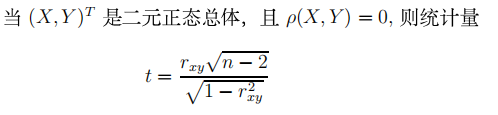服从自由度为n-2的t分布
利用此分布的性质，可以对数据X和Y的相关性进行检验，该方法称为Pearson相关性检验。
此外，还有Spearman秩检验和Kendall秩检验，R软件中的cor.test()提供了这三种检验方法。
cor.test()使用方法
cor.test(x,y,
alternative = c("two.sided","less","spearman"),
method = c("pearson","kendall","spearman"),
exact = NULL, conf.level = 0.95,...)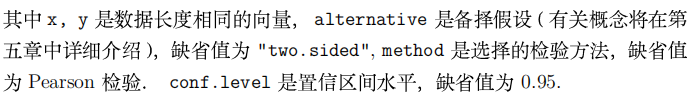另一种使用格式：
cor.test(formula, data, subset, na.action, ...)

多元数据的数字特征及相关矩阵
关于相关性检验，R软件没有为多元数据提供更多的函数，仍是cor.test（）作两两分量的相关性检验
例：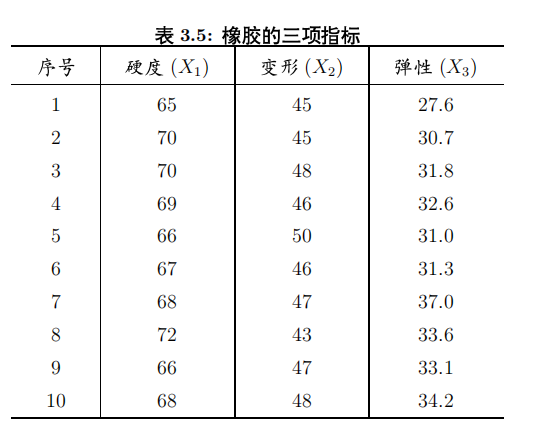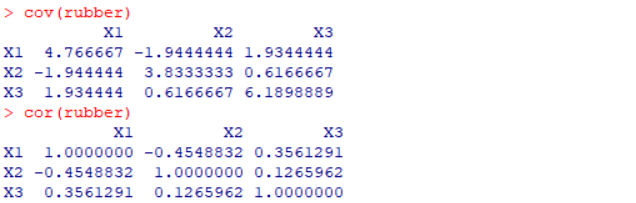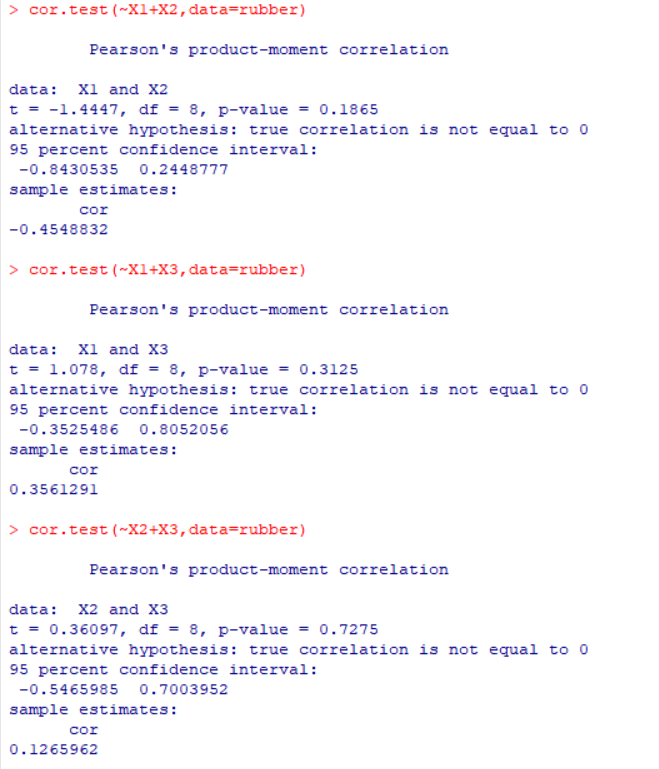从计算结果可以看出，X1,X2,X3两两均是不相关的
多元数据的图表示方法
轮廓图
outline<-function(x, txt=TRUE){
# x is a matrix or data frame of data
if (is.data.frame(x)==TRUE)
x<-as.matrix(x)
m<-nrow(x); n<-ncol(x)
plot(c(1,n), c(min(x),max(x)), type="n",
main="The outline graph of Data",
xlab="Number", ylab="Value")
for(i in 1:m){
lines(x[i,], col=i)
if (txt==TRUE){
k<-dimnames(x)[][i]
text(1+(i-1)%%n, x[i,1+(i-1)%%n], k)
}
}
}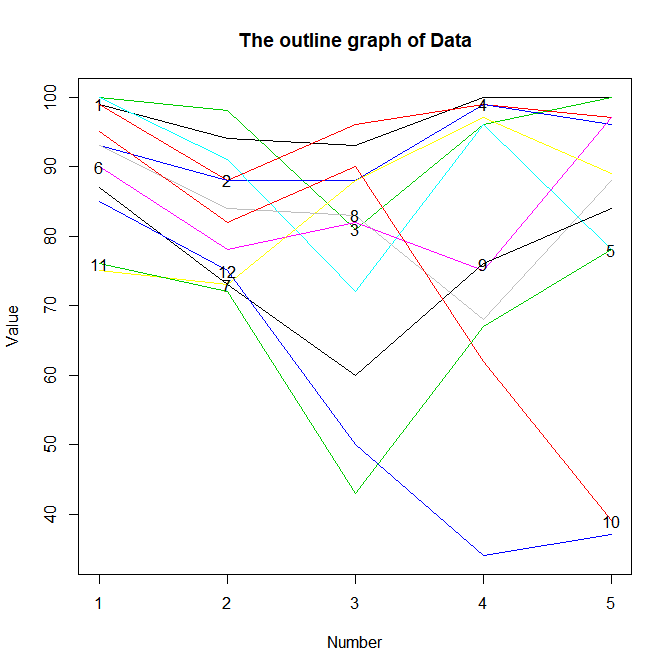星图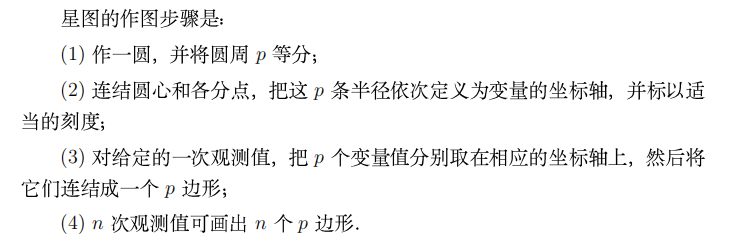R软件中给出了作星图的函数stars（）
stars(x)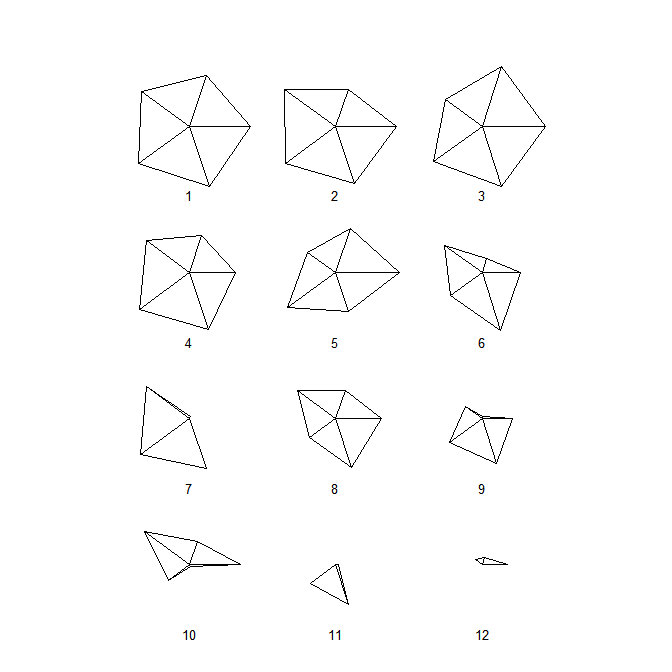调整stars中的参数：
stars(x, full=FALSE, draw.segments = TRUE,
key.loc = c(5,0.5), mar = c(2,0,0,0) )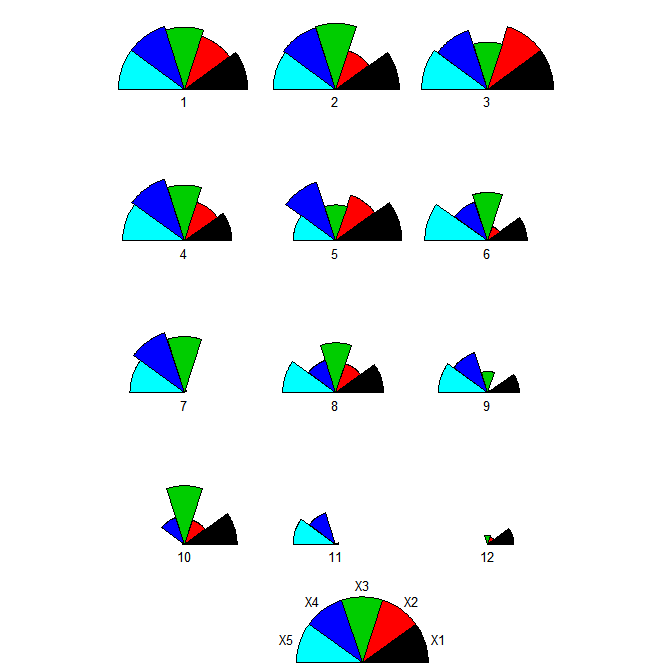调和曲线图
调和曲线图是Andrews提出来的三角表示法，其思想是将多维空间中的一个点对应于二维平面的一条直线，对于p维数据，假设X_r是第r观测值，即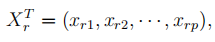则对应的调和曲线是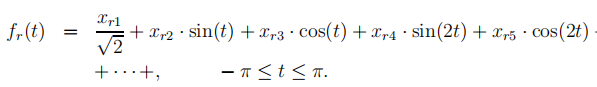n次观测数据对应n条曲线，在同一张纸上就是一张调和曲线图，当各变量数据的数值相差太大时，先标准化再画图。
按照上述计算式，编写调和曲线函数：
unison<-function(x){
# x is a matrix or data frame of data
if (is.data.frame(x)==TRUE)
x<-as.matrix(x)
t<-seq(-pi, pi, pi/30)
m<-nrow(x); n<-ncol(x)
f<-array(0, c(m,length(t)))
for(i in 1:m){
f[i,]<-x[i,1]/sqrt(2)
for( j in 2:n){
if (j%%2==0)
f[i,]<-f[i,]+x[i,j]*sin(j/2*t)
else
f[i,]<-f[i,]+x[i,j]*cos(j%/%2*t)
}
}
plot(c(-pi,pi), c(min(f),max(f)), type="n",
main="The Unison graph of Data",
xlab="t", ylab="f(t)")
for(i in 1:m) lines(t, f[i,] , col=i)
}

unison（x）得到图像如下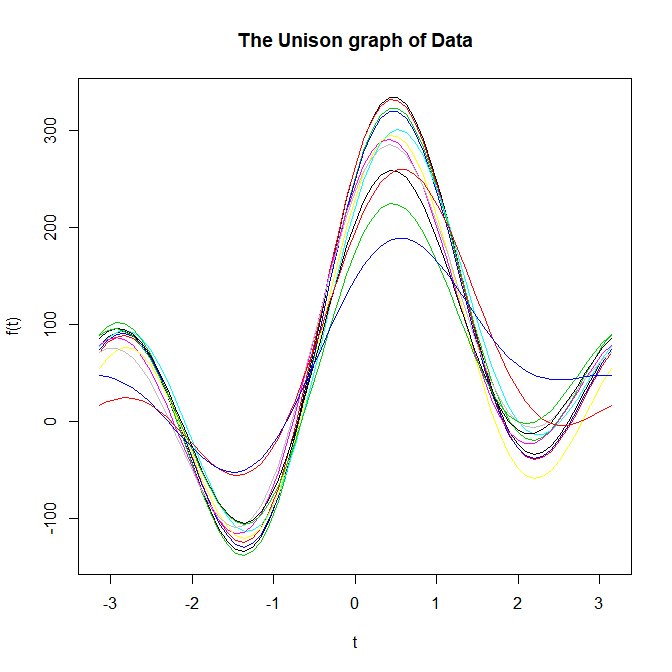展开全文r语言 统计学
• 统计：在我的理解里面，是对各种数字，情况的一个汇总，就像我们每天做的表格一样，汇集了不同种了不同数量不同来源的数据，但这些数据杂乱无章怎么识别，那么就需要一个方法来描述这些数据——描述统计分析，就是让...
统计：在我的理解里面，是对各种数字，情况的一个汇总，就像我们每天做的表格一样，汇集了不同种了不同数量不同来源的数据，但这些数据杂乱无章怎么识别，那么就需要一个方法来描述这些数据——描述统计分析，就是让数据可视化简洁化，让人们有需求的对数据进行分析和解读。描述统计四个指标：1.平均值生活中最经常碰到的一种指标，但出现异常数据的时候，容易造成分析错误。2.四分位数定义：是指在统计学中把所有数值由小到大排列并分成四等份，处于三个分割点位置的数值，处于25%的位置为下分位点，处于75%的位置为上分位点。特点：可以识别异常的数据加以核实及删改。识别异常数据方法：最小估计值：Q1-k(Q3-Q1)最大估计值：Q3-k(Q3-Q1)k=1.5 中度异常k=3极度异常（Q1为下分位点，Q2为上分位点）3.标准差标准差是对整体数据的波动大小进行判断。标准差公式：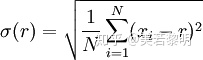标准差在不同的应用中有不同的额判断方式：例如：一个球员是否值得留在球队，可分析其每场比赛得分的标准差，判断是否稳定，标准差越小越好，对于一个刚成立的公司来说，需分析其后期是否有较大的增值空间，则其正向波动越大则越好。4.标准分表示某个数值距离平均值有多少个标准差公式：【Z=(X-X_bar)/S式中，X为原始分数，X_bar为原始分的平均数，S为原始分的标准差。】等于0，等于平均值；大于零，大于平均值；小于零，小于平均值。数据集的选择：表1购买商品数据集表1购买商品（sample)sam_tianchi_mum_baby_trade_history.csv）1）表1购买商品字段用户ID：user_id 是用户注册的ID号，可识别是否为同一个人产生的购买行为商品编号（item_id）：auction_id，用于对应商品名称商品二级分类：cat_id，商品种类ID，表示商品属于哪个类别商品一级分类：cat1，商品种类ID，表示商品属于哪个类别这两个分为一个大类，一个小类，可分析哪类产品销量比较高，销售力度大。商品属性:property，可分析哪种类别的产品购买需求量大，对于商家来说可以增加相关产品库存量购买数量：buy_mount：可分析相关产品销量的多少购买时间:day 可分析在什么时间段内用户购买量最多你想从该数据集中得到哪些描述统计信息？1.购买数量的平均值，四分位数，标准差2.购买时间的标准差从该数据集中分析哪些业务问题？1.用户在哪些时间段内购买需求最大，字段需求：购买时间:day，购买数量：buy_mount2.哪种商品编号购买量， 字段需求：最多商品编号（item_id）：auction_id，购买数量：buy_mount3.该商品编号的商品哪种属性的商品购买量最多，字段需求：商品编号（item_id）：auction_id，商品属性:property，购买数量：buy_mount还需要学习的技能：标准差四分位数在Excel的函数公式，Python箱线使用
展开全文• 写在前面什么是描述统计分析，这不仅是数据分析面试中最常见的问题，也是我们日常分析中必过的一道关卡。要把握数据的分布特征，需要从3个方面进行描述：集中趋势、离散程度和分布形状。1、集中趋势...


写在前面什么是描述性统计分析，这不仅是数据分析面试中最常见的问题，也是我们日常分析中必过的一道关卡。要把握数据的分布特征，需要从3个方面进行描述：集中趋势、离散程度和分布形状。1、集中趋势 集中趋势就是指一组数据向某一中心靠拢的程度，通常有以下指标：众数对分类数据来说，众数是最适合描述其集中趋势的值，它是指一组数据中出现次数最多的变量，众数不受极端值的影响，但是可能不唯一，且当数据量较多时，才有意义。中位数对数据型数据来说，可以用中位数来描述其集中趋势的度量，中位数就是将一组数据排序以后处于中间位置的值，那么如何寻找中间位置呢？1、 将这组数据按顺序排列2、 中间数值所在的位置是(n+1)/2，n表示n个数3、 当n为奇数时，中间位置的数就是中位数，当n为偶数时，中间位置两个数的均值为中位数。显然这里有两种情况，当n为奇数和偶数两种情况，求下面这组数据的中位数0 1 2 3 4 5 6 7 8这里n=9，中位数的位置=(9+1)/2 = 5，那么中位数就是4.0 1 2 3 4 5 6 7 8 9当n=10时，中位数的位置是 (10+1)/2 = 5.5，那么中位数就是第5个位置的数和第6个位置的数的平均数，也就是(4+5)/2 = 4.5知道计算的原理，但其实不用我们自己去算，软件中有相应的函数，如Excel中的median函数可以计算中位数。中位数也不受极值的影响。四分位数同样，四分位数也是对数值型数据集中趋势的度量，后面的度量都适用于数值型数据。四分位数就是将数据排序后四等分，处于25%（下四分位数）和75%（上四分位数）位置上的值即四分位数，箱型图可以很好地用来描述四分位数。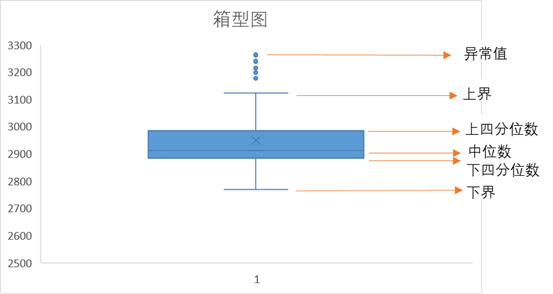Excel中可以用quartile函数来计算四分位数。平均数简单平均数：就是算术平均数，所有数值相加再除以总个数。加权平均数：数据分组后每组有相应的权重。几何平均数：n个变量乘积的n次方根，主要用于计算平均比率，如增长率的平均值。Excel中用average函数计算平均数。如果分布是对称的，那么众数=中位数=平均数，如果数据左偏，那么平均数<中位数<众数，如果数据右偏，那么众数<中位数<平均数，根据数据的分布形态，选择适合的集中趋势度量去描述。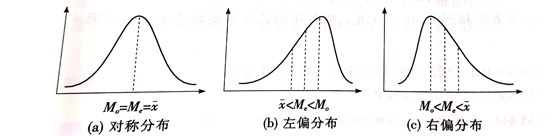2、离散程度 离散程度就是描述各个变量远离其中心值的程度，通常有以下指标：异众比率指非众数组的频数占总频数的比例，较多地用在分类数据中，用来体现众数的代表性。异众比率越大，说明非众数组占比越大，那么众数就不能很好地代表这组数据。四分位差对于数值型数据，四分位差可以来描述数据的离散程度，四分位差就是上下四分位数的差。反映了中间50%数据的离散程度，四分位差越小，说明中间的数据越集中，极差极差就是最大值与最小值的差，容易受极值的影响。平均差各变量与其平均数离差绝对值的平均数，反映了每个数与平均数的差异程度,Excel里用avedev计算平均差。方差和标准差对数值型数据，怎么能少得了我们熟知的方差和标准差。方差就是各变量与其平均数离差平方的平均数，方差的平方根就是标准差，方差和标准差是应用最广的描述数据离散程度的度量。Excel里用stdev来计算标准差。3、分布形状 描述分布形状的度量有偏态和峰态偏态数据分布对称性的描述，统计量是偏度，偏度>0，则频数分布的高峰向左偏移，呈右（正）偏态分布；偏度<0，则频数分布的高峰向右偏移，呈左（负）偏态分布；|偏度|>1，呈高度偏态，0.5<|偏度|<1，呈中等偏态，一个记忆方法就是，尾巴在哪边就是哪偏。对于偏态，在之前的文章 中也有写过。如何处理偏态数据？峰态是描述数据平峰或尖峰程度的度量。统计量是峰度。同偏态一样，峰态也是与标准正态分布来比较的，峰度>0，数据分布更集中，为尖峰分布，峰度<0，数据分布更分散，为平峰分布。以上提到的各种统计量除了用Excel中的函数来计算外，还可直接用【数据分析】功能中的【描述统计】命令直接得到。猜你喜欢:简单地聊聊统计学如何进行数据图形化？什么是好的数据指标：精益数据分析泰坦尼克号数据分析深入浅出数据分析数据分析都有哪些岗位？展开全文数据挖掘 统计学 数据可视化 人工智能
• 数据分析 1 统计学简介 1.1 统计学简介 统计学(Statistics): 收集、处理、分析、解释数据并从数据中得出结论的科学。...推断统计其实是建立在描述统计基础上，对总体数据有了大致了解后，运用一些分析方法，对
• 【R描述统计分析】绘图命令高水平绘图函数plot()函数显示多变量数据显示图形实例1：山区地貌图实例2：绘制等值线图和三维曲面图低水平作图函数加点与线的函数在点处加标记在图上加直线 高水平绘图函数 高水平作图...r语言 统计学
• 简单描述统计分析 一、 means过程 （一）例题和语句分析 例题1：某车间有30个人分成4组，求车间工人平均每小时制作的配件个数 data data3_1; input no w n; /*按自由格式输入变量no、w和n*/ cards; 01 10 35 02 ...
• 1、学习数据分析有什么意义？有一个很典型的例子我跟大家分享一下。...2、什么是描述统计分析？用关键数据描述数据集的整体情况平均值：在一组数据中所有数据之和再除以这组数据的个数，表示一组数...
• 【R描述统计分析】数据的分布分布函数正态分布Possion分布其他分布函数或分布律图形二项分布泊松分布几何分布超几何分布负二项分布分布图直方图与核密度估计函数经验分布正态QQ图茎叶图箱线图五数总括 分布函数 正态...r语言 统计学
• 数据的描述统计量 33:《数据分析基础》的复习笔记3 主要涉及用R语言求解 1. 描述水平的统计量 （1）平均数 （2）分位数 ①中位数 ②四分位数 ③百分位数 （3）众数 2.描述差异的统计量 （1）极差和四分之位差 ...
• 什么是描述统计分析将一系列复杂的数据减少到几个能起到关键作用的数据，用这些有代表性的数字来代表数据集。（关键点就在于找到关键的数据来描述数据集的整体情况）二.描述数据常用的4个指标平均值： 含义：表示一...
• 什么是描述统计分析，这不仅是数据分析面试中最常见的问题，也是我们日常分析中必过的一道关卡。 要把握数据的分布特征，需要从3个方面进行描述：集中趋势、离散程度和分布形状。 1、集中趋势 集中趋势就是指一...集中趋势 统计学
• 点击蓝字关注我们SPSS【分析】菜单下的描述统计中包括了一系列基本统计分析过程，如常用的频数分布表、描述统计量、交叉列联表及其独立性建议以及探索分析等。当得到审核无误的原始数据后，需要认识数据、了解数据...
• （一）描述数据常用的4个指标 1.平均值 计算简单，但是易受异常值、极端值的影响 2.四分位数 2.1中位数 1）将数字从小到达排序 2）中间位置：奇数即为中间的数，偶数为中间两个数的平均值 2.2四分位数 上界：数据中...大数据 python 数据挖掘
• 我们采用IBM SPSS23.0作为数据挖掘的操作软件。...1.基本描述统计分析我们准备采用数据表与饼图相结合的方式，呈现数据的基本概况，使人们对留学生汉语辞书使用与需求的基本现状有一个总体和直观的了解。...
• 写在前面什么是描述统计分析，这不仅是数据分析面试中最常见的问题，也是我们日常分析中必过的一道关卡。要把握数据的分布特征，需要从3个方面进行描述：集中趋势、离散程度和分布形状。1、集中趋势集中趋势就是指...
• 一、描述统计分析概念 描述性统计，是指运用制表和分类，图形以及计算概括性数据来描述数据特征的各项活动。描述统计分析要对调查总体所有变量的有关数据进行统计性描述，主要包括数据的频数分析、集中趋势分析...
• 写在前面什么是描述统计分析，这不仅是数据分析面试中最常见的问题，也是我们日常分析中必过的一道关卡。要把握数据的分布特征，需要从3个方面进行描述：集中趋势、离散程度和分布形状。1、集中趋势集中趋势就是指...java平均差...